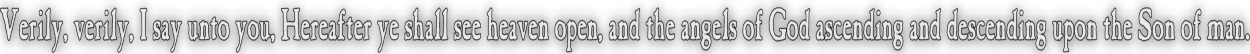None:
Polyps:
Strongs:CreationismNaive Set Theory Naive set theory is perhaps the most familar form of sets introduced to pupils at school, that sets are a collection of items, usually arranged with relations that show some commonality that describes the members of that set. The operations of intersection and union upon sets can be described in terms of OR and AND. A ∪ B = {x : x is in either A or B} A ∪ B = {x : X is in both A and B} We also have the symmetric difference A v B = {x : x is in A\B or B\A but not A ∩ B} Where A \ B = A - B = {x : x is in A and not B} clearly A v B = (A ∪ B) - (A ∩ B) We may also add sets, so A + B = (A ∪ B) + (A ∩ B) = {x, y : x in A, y in B} These simple rules define the behaviour that most people have come to expect from what is regarded as "naive" set theory. In terms of logic, "v", the symmetric difference takes the place or logical "XOR" and "union" "OR", and intersection "AND" The operation of "NOT" requires a "set-space" usually referred to in Naive set theory as "omega" so that NOT(A) = omega - A. Omega is usually thought of as a "set of everything" but such sets are actually fraught with paradoxes. It makes more sense to define what is meant by the set-space omega before including these rules as above. Logic has its own language, but the set space is often defined axiomatically: that "omega" is simply "The set of all provable statements arising from this finite set of axioms". The dialectic Then has A ∪ B = omega - (A ∩ B), a very strange idea. We would not expect this naively at all! Perhaps the union is omega and A and B disjoint? A and B would then be logical opposites and the system would be "thesis/antithesis" Similarly then, we may have omega = A + B, the sum of the two sets ! Then however, we have counted some elements (in A ∩ B) twice. We immediately recover a need for the "great sword" of the second seal, as if the intersection cancels itself out (* see below) and we obtain the statement A v B, as it were "XOR". The dialectic has the 'wine' as 'w' and the set S as the synthesis if that we may write A = {oil, x} and B = {oil,y} (w + A) * (w + B) = w + (A v B) = S Where '*' is the application of the dialectic. Likewise we may also write using our previous elements {d,e,f,g} that A = {d,e,f} = g B = {d,e,g} = f as wine = 'd', oil = 'e' S = (A*B) = d + ((A\d) v (B\d)) or S \ d = (A \ d) v (B \ d) or even A v B = S \ d S = (A v B) + d Of course if 'd' and the others are also triples, then A = {d,e,f} = {{e,f,g},{d,f,g},{d,e,g}} B = {d,e,g} = {{e,f,g},{d,f,g},{d,e,f}} wine = d = {e,f,g} oil = e = {d,f,g} S = {{e,f,g},{{d,e,g},{d,e,f}} = (A v B) + d as before. However we can parse the list with summation or counting and find: S = {{e,f,g},{{d,e,g},{d,e,f}} = {3e, 2d, 2f, 2g} And if every element cancels out as "order 2" or that "2x = nothing", 3y = y etc. S = e = {d,f,g} remains as before. However if we add e = {d,f,g} to itself we would have 2e = {3e, 3d, 3f, 3g} = (nothing) = {d,e,f,g} so our "set space" is somehow null. Clearly countng may mess with the mind. What we have from (*) is a spherical system of sorts, The thesis/antitheses divide the sphere into two "hemispheres", the negation of a set is actually itself: (2x = null) and the set space omega is simultaneously the intersection of A and B (empty as the intersection of both hemispheres) as well as the sum, the whole sphere. Omega, also equal to its negation is also the empty set or "point" on the sphere, and the intersection of A and B that partition the sphere is such that the empty intersection (a point) becomes the equivalent of the sum A+B where A and B are disjoint. Of course, the devil is in the details, There are possibilities of omega being both an open and a closed set! Could this be related to the colouring of any map with only four colours? Maybe, If you have any ideas, please let me know! Continue To Next Page Return To Section Start Return To Previous Page'